• 异或运算的 BP算法解决 智能优化方法 技术报告 题目基于 BP算法的异或运算的解决 班 级 信科 13-01 班 学 号 08133367 姓 名 张谊坤 任课教师 姚 睿 2 目 录 一 摘 要 3 二 技术路线及实现方法 2.1 输 入 层 和 输 ...
• 主要介绍了Java编程实现对十六进制字符串异或运算代码示例，简述了异或运算以及具体实例，具有一定借鉴价值，需要的朋友可以参考下。
• 可进行十六进制字符串按位异或运算，就是输入十六进制数，每个十六进制数间输入空格，然后点输出即可得到结果
• 交换两个数的值，通常用利用一个变量来交换数值，异或交换两个数的值是资源开销最小的方法，不需要中介数，原理简单的来说就是异或的负负得正
• 运算运算分量只能是整型或字符型数据，位运算运算对象看作是由二进位组成的位串信息，按位完成指定的运算，得到位串信息的结果
• 异或运算，传入两个数据进行异或运算。得到相应的数据
• 主要为大家详细介绍了java实现两个文件的异或运算，具有一定的参考价值，感兴趣的小伙伴们可以参考一下
• ## 异或运算

千次阅读 2020-02-17 01:00:53
异或运算法则 如果a、b两个值不相同，则异或结果为1。如果a、b两个值相同，异或结果为0。异或也叫半加运算，其运算法则相当于不带进位的二进制加法：二进制下用1表示真，0表示假，则异或的运算法则为：0⊕0=0，1⊕0...
异或运算法则
如果a、b两个值不相同，则异或结果为1。如果a、b两个值相同，异或结果为0。异或也叫半加运算，其运算法则相当于不带进位的二进制加法：二进制下用1表示真，0表示假，则异或的运算法则为：0⊕0=0，1⊕0=1，0⊕1=1，1⊕1=0（同为0，异为1），这些法则与加法是相同的，只是不带进位，所以 异或常被认作不进位加法。（来源于搜狗百科）
例如，计算 1011101^1000011: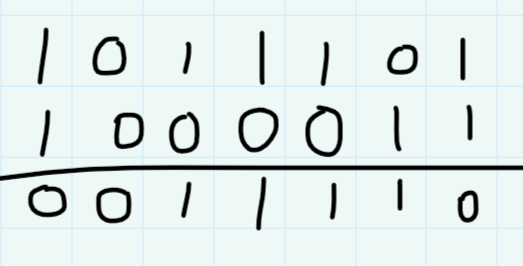异或性质与扩展
（用不进位相加较好理解）
0 ^ N = NN ^ N = 0异或运算满足交换律和结合律 c =a ^ b =b ^ a c =（ a ^ b ）^ c = a ^ ( b ^ c )不用额外变量交换两个数：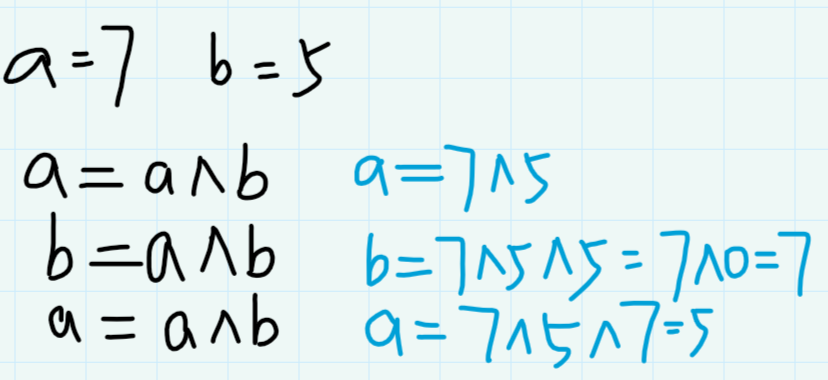一个数组中有一种数出现了奇数次，其他数都出现了偶数次，怎么找到这个数？ 例如，该数组为 a[]={1, 2, 2, 3, 3} 将所有的数全部异或运算，运算结果就是出现了奇数次的数。一个数组中有两种出现了奇数次，其他数都出现了偶数次，怎么找到这两个数？ 例如，该数组为a[]={1,2,4,4,5,5}
让该数组中所有的数字做异或运算，那么设结果 eor == a ^ b != 0 ;因为eor 不为 0 ，则可以假设 a 与 b在某位上，比如在第三位上，a第三位是1，则b的第三位为0；在其余的出现偶数次的数字中，找出所有在第三位为1的数；用变量 eor’ 与这个数组中所有第三位为1的数做异或运算 ，则 eor’ 最终的答案为a，因为所有第三位为1的数字，除了a，其余为偶数个，异或运算后为0。(因为除了a 与 b ，其余数字的个数都为偶数个，那么可以确定第三位为1的和第三位为0的个数都为偶数个。因为 eor = a ^ b,且其余偶数运算之后结果为0，如果第三位为1的数字个数为奇数，那么第三位为0的数字个数也为奇数，那么将他们全部进行异或运算后，第三位数字为1，不为0，与实际不符。)再用eor与eor’做异或运算，即a ^ b ^ a = b, 再计算 eor ^ eor’ ^ eor ,即 b ^ a ^ b = a;
以下给出该题解的代码：首先了解如何取到该数最右端的1，就是该数取反加一再与该数 （核心：int rightOne = eor & ( ~ eor + 1 ); ）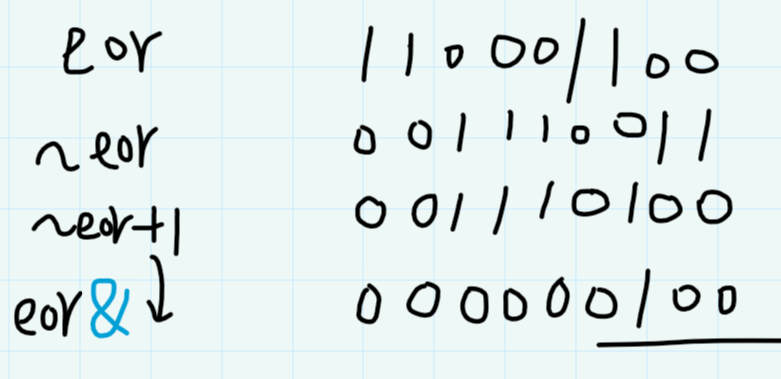public static void printOddTimesNum(int[] arr){
int eor=0;
for(int i=0;i<arr.length;i++)
eor^=arr[i];
//提取出最右端的1
int rightOne=eor&(~eor+1);

int onlyOne=0; //eor'
//找出这个数组中所有在最右端为1的数字，做异或运算
for(int cur:arr){
if((cur&rightOne)!=0)
//说明该数当前位上的数字也是1
{
onlyOne^=cur;
}
}
System.out.println(onlyOne+" "+(eor^onlyOne));
}

展开全文• 什么是异或_异或运算异或运算的作用 异或，是一个数学运算符，英文为exclusive OR，缩写为xor，应用于逻辑运算。异或的数学符号为“⊕”，计算机符号为“xor”。其运算法则为： 　a⊕b = （¬a ∧ b） ∨ （a ∧...
什么是异或_异或运算及异或运算的作用
异或，是一个数学运算符，英文为exclusive OR，缩写为xor，应用于逻辑运算。
异或的数学符号为“⊕”，计算机符号为“xor”。其运算法则为：
a⊕b = （¬a ∧ b） ∨ （a ∧¬b）
如果a、b两个值不相同，则异或结果为1。如果a、b两个值相同，异或结果为0。
异或也叫半加运算，其运算法则相当于不带进位的二进制加法：
二进制下用1表示真，0表示假，则异或的运算法则为：0⊕0=0，1⊕0=1，0⊕1=1，1⊕1=0（同为0，异为1），
这些法则与加法是相同的，只是不带进位。
异或略称为XOR、EOR、EX-OR
程序中有三种演算子：XOR、xor、⊕。
使用方法如下
z = x ⊕ y
z = x xor y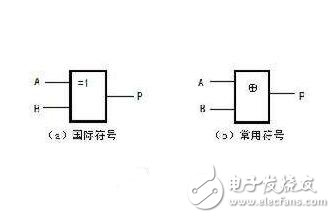异或运算的作用
参与运算的两个值，如果两个相应bit位相同，则结果为0，否则为1。
即：
0^0 = 0，
1^0 = 1，
0^1 = 1，
1^1 = 0
按位异或的3个特点：
（1） 0^0=0，0^1=1 0异或任何数＝任何数
（2） 1^0=1，1^1=0 1异或任何数－任何数取反
（3） 任何数异或自己＝把自己置0
按位异或的几个常见用途：
（1） 使某些特定的位翻转
例如对数10100001的第2位和第3位翻转，则可以将该数与00000110进行按位异或运算。
10100001^00000110 = 10100111
（2） 实现两个值的交换，而不必使用临时变量。
例如交换两个整数a=10100001，b=00000110的值，可通过下列语句实现：
a = a^b； 　　//a=10100111
b = b^a； 　　//b=10100001
a = a^b； 　　//a=00000110
（3） 在汇编语言中经常用于将变量置零：
xor a，a
（4） 快速判断两个值是否相等
举例1： 判断两个整数a，b是否相等，则可通过下列语句实现：
return （（a ^ b） == 0）
{
}
可以利用按位异或实现快速比较， 最新的实现已经修改为：
{
}
5 应用通式：
对两个表达式执行按位异或。
result = expression1 ^ expression2
参数
result
任何变量。
expression1
任何表达式。
expression2
任何表达式。
说明
^ 运算符查看两个表达式的二进制表示法的值，并执行按位异或。该操作的结果如下所示：
0101 （expression1）1100 （expression2）----1001 （结果）当且仅当只有一个表达式的某位上为 1 时，结果的该位才为 1。否则结果的该位为 0。
只能用于整数
下面这个程序用到了“按位异或”运算符：
class E
{ public staTIc void main（String args［ ］）
{
char a1=‘十’ ， a2=‘点’ ， a3=‘进’ ， a4=‘攻’ ;
char secret=‘8’ ;
a1=（char） （a1^secret）;
a2=（char） （a2^secret）;
a3=（char） （a3^secret）;
a4=（char） （a4^secret）;
System.out.println（“密文：”+a1+a2+a3+a4）;
a1=（char） （a1^secret）;
a2=（char） （a2^secret）;
a3=（char） （a3^secret）;
a4=（char） （a4^secret）;
System.out.println（“原文：”+a1+a2+a3+a4）;
}
}
就是加密啊解密啊
char类型，也就是字符类型实际上就是整形，就是数字。
计算机里面所有的信息都是整数，所有的整数都可以表示成二进制的，实际上计算机只认识二进制的。
位运算就是二进制整数运算啦。
两个数按位异或意思就是从个位开始，一位一位的比。
如果两个数相应的位上一样，结果就是0，不一样就是1
所以111^101=010
那加密的过程就是逐个字符跟那个secret字符异或运算。
解密的过程就是密文再跟同一个字符异或运算
010^101=111
至于为什么密文再次异或就变原文了，这个稍微想下就知道了。。
异或运算：按位异或运算符
首先异或表示当两个数的二进制表示，进行异或运算时，当前位的两个二进制表示不同则为1相同则为0.该方法被广泛推广用来统计一个数的1的位数！
参与运算的两个值，如果两个相应bit位相同，则结果为0，否则为1。
即：
0^0 = 0，
1^0 = 1，
0^1 = 1，
1^1 = 0
按位异或的3个特点：
（1） 0^0=0，0^1=1 0异或任何数＝任何数
（2） 1^0=1，1^1=0 1异或任何数－任何数取反
（3） 任何数异或自己＝把自己置0
按位异或的几个常见用途：
（1） 使某些特定的位翻转
例如对数10100001的第2位和第3位翻转，则可以将该数与00000110进行按位异或运算。
10100001^00000110 = 10100111
（2） 实现两个值的交换，而不必使用临时变量。
例如交换两个整数a=10100001，b=00000110的值，可通过下列语句实现：
a = a^b； 　　//a=10100111
b = b^a； 　　//b=10100001
a = a^b； 　　//a=00000110
位运算
位运算时把数字用二进制表示之后，对每一位上0或者1的运算。理解位运算的第一步是理解二进制。二进制是指数字的每一位都是0或者1.比如十进制的2转化为二进制之后就是10。
其实二进制的运算并不是很难掌握，因为位运算总共只有5种运算：与、或、异或、左移、右移。如下表：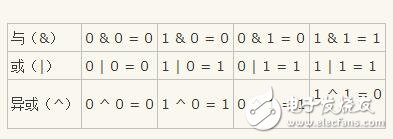左移运算：
左移运算符m《《n表示吧m左移n位。左移n位的时候，最左边的n位将被丢弃，同时在最右边补上n个0.比如：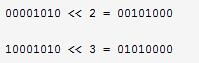右移运算：
右移运算符m》》n表示把m右移n位。右移n位的时候，最右边的n位将被丢弃。但右移时处理最左边位的情形要稍微复杂一点。这里要特别注意，如果数字是一个无符号数值，则用0填补最左边的n位。如果数字是一个有符号数值，则用数字的符号位填补最左边的n位。也就是说如果数字原先是一个正数，则右移之后再最左边补n个0；如果数字原先是负数，则右移之后在最左边补n个1.下面是堆两个8位有符号数作右移的例子：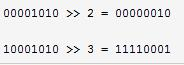关于移位的运算有这样的等价关系：把整数右移一位和把整数除以2在数学上是等价的。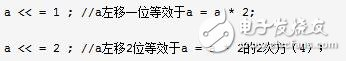计算机内部只识别1、0，十进制需变成二进制才能使用移位运算符《《，》》 。
int j = 8;
p = j 《《 1;
cout《《p《《endl;
在这里，8左移一位就是8*2的结果16 。
移位运算是最有效的计算乘/除乘法的运算之一。
按位与（&）其功能是参与运算的两数各对应的二进制位相与。只有对应的两个二进制位均为1时，结果位才为1，否则为0 。参与运算的数以补码方式出现。
先举一个例子如下：
题目：请实现一个函数，输入一个正数，输出该数二进制表示中1的个数。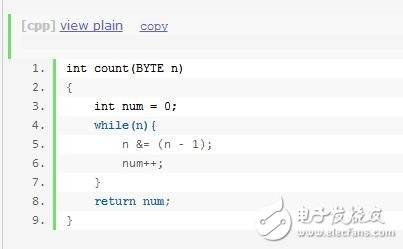这里用到了这样一个知识点：把一个整数减去1，再和原整数做与运算，会把该整数最右边一个1变成0 。 那么一个整数的二进制表示中有多少个1，就可以进行多少次这样的操作。
总结：把一个整数减去1之后再和原来的整数做位与运算，得到的结果相当于是把整数的二进制表示中的最右边一个1变成0 。
位运算的应用可以运用于很多场合：
举例：输入两个整数m和n，计算需要改变m的二进制表示中的多少位才能得到n。
解决方法：第一步，求这两个数的异或；第二步，统计异或结果中1的位数。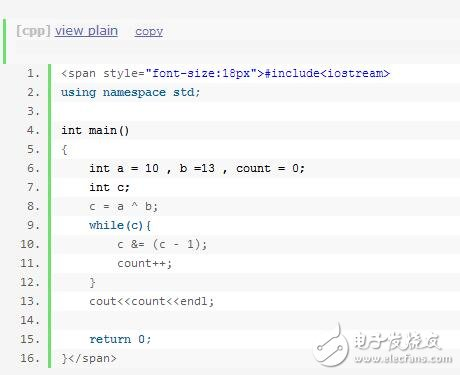接下来我们再举一例，就可以更好的说明移位运算了：用一条语句判断一个整数是不是2的整数次方。
解决方法：一个整数如果是2的整数次方，那么它的二进制表示中有且只有一位是1，而其它所有位都是0 。 根据前面的分析，把这个整数减去1后再和它自己做与运算，这个整数中唯一的1就变成0了。
解答：！（x & （x - 1））
展开全文• “一个整型数组里除了两个数字之外，其他的数字都出现了两次。请写程序找出这两个只出现一次的数字？”这是经典的算法题，乍看这个题的思路特别多。
• 适合在校大学生初步实验使用，将此程序直接粘贴到Matlab的m文件中，便可直接运行，得到结果，本人已在博客中发表“与”运算实验，神经网络网址如...通过看我的博文，自己编出“异或运算代码
• 大家比较熟悉的逻辑运算，主要是"与运算"（AND）和"或运算"（OR），还有一种"异或运算"（XOR），也非常重要。 本文介绍异或运算的含义和应用。
目录
1 含义
2 运算定律
3 应用
3.1 简化计算
3.2 交换值
3.3 加密
3.4 数据备份
4 一道面试题
5 python的异或

大家比较熟悉的逻辑运算，主要是"与运算"（AND）和"或运算"（OR），还有一种"异或运算"（XOR），也非常重要。
本文介绍异或运算的含义和应用。
1 含义
XOR 是 exclusive OR 的缩写。英语的 exclusive 意思是"专有的，独有的"，可以理解为 XOR 是更单纯的 OR 运算。异或的数学符号为“⊕”，计算机符号为“eor”。
我们知道，OR 运算的运算子有两种情况，计算结果为true。
（1）一个为 true，另一个为 false;
（2）两个都为 true。
上面两种情况，有时候需要明确区分，所以引入了 XOR。
XOR 排除了第二种情况，只有第一种情况（一个运算子为true，另一个为false）才会返回 true，所以可以看成是更单纯的 OR 运算。也就是说， XOR 主要用来判断两个值是否不同。

其运算法则为：
a⊕b = (¬a ∧ b) ∨ (a ∧¬b)
如果a、b两个值不相同，则异或结果为1。如果a、b两个值相同，异或结果为0。异或也叫半加运算，其运算法则相当于不带进位的二进制加法：二进制下用1表示真，0表示假，则异或的运算法则为：0⊕0=0，1⊕0=1，0⊕1=1，1⊕1=0（同为0，异为1），这些法则与加法是相同的，只是不带进位，所以异或常被认作不进位加法。
XOR 一般使用插入符号（caret）^表示。如果约定0 为 false，1 为 true，那么 XOR 的运算真值表如下。
0 ^ 0 = 0
0 ^ 1 = 1
1 ^ 0 = 1
1 ^ 1 = 0
2 运算定律
XOR 运算有以下的运算定律。由于非常简单，这里就省略证明了。
（1）归零律：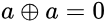一个值与自身的运算，总是为 false。
x ^ x = 0
（2）恒等律：一个值与 0 的运算，总是等于其本身。
x ^ 0 = x
（3）交换律：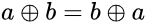可交换性
 x ^ y = y ^ x
（4）结合律：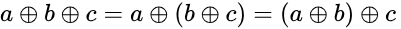结合性
x ^ (y ^ z) = (x ^ y) ^ z
（5）自反：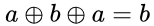（6）d = a ⊕ b ⊕ c 可以推出 a = d ⊕ b ⊕ c.
（7）若x是二进制数0101，y是二进制数1011；
则 x ⊕ y=1110。
只有在两个比较的位不同时其结果是1，否则结果为0
即“两个输入相同时为0，不同则为1”。
3 应用
根据上面的这些运算定律，可以得到异或运算的很多重要应用。
3.1 简化计算
多个值的异或运算，可以根据运算定律进行简化。
a ^ b ^ c ^ a ^ b
= a ^ a ^ b ^ b ^ c
= 0 ^ 0 ^ c
= c
3.2 交换值
两个变量连续进行三次异或运算，可以互相交换值。
假设两个变量是 x和 y，各自的值是 a和 b。下面就是 x和 y进行三次异或运算，注释部分是每次运算后两个变量x和 y的值。
x = x ^ y // (a ^ b, b)
y = x ^ y // (a ^ b, a ^ b ^ b) => (a ^ b, a)
x = x ^ y // (a ^ b ^ a, a) => (b, a)
这是两个变量交换值的最快方法，不需要任何额外的空间。
3.3 加密
异或运算可以用于加密。
第一步，明文（text）与密钥（key）进行异或运算，可以得到密文（cipherText）。
text ^ key = cipherText
第二步，密文与密钥再次进行异或运算，就可以还原成明文。
cipherText ^ key = text
原理很简单，如果明文是 x，密钥是 y，那么 x 连续与 y 进行两次异或运算，得到自身。
(x ^ y) ^ y
= x ^ (y ^ y)
= x ^ 0
= x
3.4 数据备份
异或运算可以用于数据备份。
文件 x 和文件 y 进行异或运算，产生一个备份文件 z。
x ^ y = z
以后，无论是文件 x 或文件 y 损坏，只要不是两个原始文件同时损坏，就能根据另一个文件和备份文件，进行还原。
x ^ z
= x ^ (x ^ y)
= (x ^ x) ^ y
= 0 ^ y
= y
上面的例子是 y 损坏，x 和 z 进行异或运算，就能得到 y。
4 一道面试题
一些面试的算法题，也能使用异或运算快速求解。
请看下面这道题。
一个数组包含 n-1 个成员，这些成员是 1 到 n 之间的整数，且没有重复，请找出缺少的那个数字。
最快的解答方法，就是把所有数组成员（A 一直到 A[n-2]）与 1 到 n 的整数全部放在一起，进行异或运算。
A ^ A ^ ... ^ A[n-2] ^ 1 ^ 2 ^ ... ^ n
上面这个式子中，每个数组成员都会出现两次，相同的值进行异或运算就会得到 0。只有缺少的那个数字出现一次，所以最后得到的就是这个值。
你可能想到了，加法也可以解这道题。
1 + 2 +  ... + n - A - A - ... - A[n-2]
但是，加法的速度没有异或运算快，而且需要额外的空间。如果数字比较大，还有溢出的可能。
下面是一道类似的题目，大家可以作为练习。（快慢指针思想？）
一个数组包含 n+1 个成员，这些成员是 1 到 n 之间的整数。只有一个成员出现了两次，其他成员都只出现一次，请找出重复出现的那个数字。
5 python的异或
让我们先来看两段对话：

技术员：这个水坝系统的设计思路为按下紧急按钮或者水位高于危险水位时，系统发出警报
提问者：这个“或者”是
排他的么？
技术员：什么意思？
提问者：就是说，按下紧急按钮并且水位高于危险水位时，会发出警报么？
技术员：当然会啦！！！
****************************************
发言者：他现在在北京或者上海
提问者：这个“或者”是排他的么？
发言者：什么意思？
提问者：就是说，他有可能在北京的同时在上海么？
发言者：这怎么可能呢？？？？？？

显然，这两段对话中的“或者”代表的意思并不相同。
第一个“或者”并不排他，也就是普通或者 在Python中用逻辑运算符 or 表示第二个“或者”是排他的，也就是我们说的异或（xor）在Python中则用 ^ 表示
让我们用真值表来看一下异或的运算逻辑：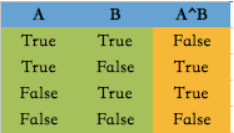也就是说：
“A B有一个为真 但不同时为真” 的运算称作异或。
如果我们将布尔值之间的异或换成数字之间的异或会发生什么？
0 ^ 0
0 ^ 1
1 ^ 0
1 ^ 1

>>>0
1
1
0
结果告诉我们 数字相同异或值为0 数字不相同异或值为1
让我们再试试0，1除外的数字：
5 ^ 3
>>>6
为什么答案会是6，不应该是1么？？这背后发生了什么？
异或是基于二进制基础上按位异或的结果 5 ^ 3 的过程 其实是将5和3分别转换为二进制：
5 = 0101(b)
3 = 0011(b)
按位异或：
0^0 ->01^0 ->10^1 ->11^1 ->0
排起来就是0110(b) 转换为十进制：6
注：可以用bin计算一个数字的二进制值。
bin(2)
# '0b10'
bin(10)
# '0b1010'
具体的用法：
本来我们想把一个flag在True和False之间转换 需要这么写：
flag = True
if something:
flag = False if flag else True
而用异或直接这么写就可以了：
flag = False
if something:
flag ^= True

异或运算 XOR 教程：http://www.ruanyifeng.com/blog/2021/01/_xor.html
That XOR Trick：https://florian.github.io/xor-trick/


展开全文• 异或运算加密，开发环境vs2013,仅支持整型数据加密，能够实现加密和解密。
• ## 详解异或运算

千次阅读 2020-11-12 22:58:50
搞不懂异或的朋友看过来，文章写得嘎嘎明白。


详解异或运算
什么是异或运算?异或运算的特质?最最最简单的异或题注意
总结

什么是异或运算?
异或运算是位运算的一种，符号为：^ 相同为0，不同为1。 容易与同或运算记混，根据异或的特性也叫 不进位相加。
异或运算的特质?

N ^ 0 = N；N ^ N = 0；异或运行满足交换律和结合律；

根据上面3个特质，个人觉得用无进位相加更便于理解了。

N ^ 0 = N
int a = 5;				//0101
int b = a ^ 0;			//0000
System.out.println(b);	//0101

位与位异或，相同为0，不相同为1，所以b的结果为5。 也就是N^0=N，任何数异或0都等于自己。

N ^ N = 0;
int a = 5;				//0101
int b = 5;				//0101
int c = a ^ b;			//0000
System.out.println(c);

两个相同的数，二进制都是一样的，所以在位与位进行异或的时候都相同，每一位的结果都是0。 所以任何数与自己异或结果都等于0。

交换律
int a = 5;
int b = 2;
int c = a ^ b;
int d = b ^ a;
System.out.println(c==b); //true

因为异或是位与位进行计算的，所以异或的顺序不重要，结果是一样的。
看一下下面的例子。
int a = 2;
int b = 4;
int c = 8;
int d = 7;
int e = a ^ b ^ c ^ d;
int f = a ^ c ^ d ^ b;

System.out.println(e == f); //true


这个例子说明了，abcd不管在什么位置，只要是异或在一起，任何位置都不影响结果。这就是异或满足交换律。 我再用二进制解释一下👇
a : 0001
b : 0100
c : 1000
d : 0111
------------
e : 1010

纵向观察，每一位进行相加，每一位的结果 不进位 ，结果就是1010。 也就是不进位相加。

结合律
int a = 2;
int b = 5;
int c = 4;
int d = (a ^ b) ^ c;
int e = a ^ b ^ c;
int f = a ^ (b ^ c);
System.out.println(d == e);	//true
System.out.println(f == e);	//true

通过交换律得知，异或的顺序不会影响结果，所以在某一些数先异或，在异或另个数的时候，也不会影响结果。这就是异或满足结合律。
最最最简单的异或题
如何不用额外变量交换两个数?
int a = 2;
int b = 5;

普通做法👇
int temp = a;
a = b;
b = temp;

这么做肯定是不对的，要求是不用额外的变量交换a、b的值。
a = a ^ b;
b = a ^ b;
a = a ^ b;

为什么呢？ 先不要把a看成2，也不要把b看成5。前面的N如果能看懂的话，在这里只需要把a看成a，把b看成b。 先看第一行的代码
a = a ^ b;

在看第二行代码
//此时的a = a ^ b
b = a ^ b;
//换言之
//b = a ^ b ^ b;

根据异或的交换律特性，先看b ^ b的部分，任何数异或自己都等于0。 这时 a ^ 0 不就等于a了吗。 再看第三行代码
//此时
//b = a ^ b ^ b;
//a = a ^ b;
a = a ^ b;
//换言之
//a = a ^ b ^ a ^ b ^ b;

根据交换律，可以先看a ^ a = 0; b ^ b = 0; 这时 a = b ^ 0 ^ 0; 这句的结果应该不用解释了。
注意
上面这个解法只适用于a和b不在同一块区域，比如在一个数组中交换位置
int[] arr = new int[]{1,2,3,4};

如果交换a与a的位置，使用了上面的方法，最后a的结果就会变成0。 需要特别注意!
总结
还有很多异或的算法，这道题不是入门，而是基础。 异或中还有很多其他的算法改「wo」日「zai」在「xue」唠「xue」！! 欢迎点赞评论交流！！展开全文算法
• Example.java异或运算（简单的加密，解密） PrintErrorAndDebug.java输出错误信息与调试信息 test.java实现两个变量的互换（不借助第3个变量）
• 异或运算详解与练习 一、异或运算讲解 按位异或 / 同或 int main() { //按二进制位展开 //按位异或: 相同为0，不同为1 //按位同或: 相同为1，不同为0 int a = 10; //二进制: 0000 1010 int b = 6; //二进制: ...java 算法 数据结构 c语言 c++
• c语言之异或运算 异或运算，计算机相关专业比较熟悉了。相同为0，不同为1.结合计算机内部的位运算，a^a=0;与本身异或是为0的。有关的知识运用到数据交互中去。 void int_swap(int *x,int *y){ *y=*x^*y;//step1 *x...c语言
• 异或运算定义&性质题目一代码执行结果特殊说明题目二 定义&性质 位运算 相同为0，不同为1 也可以记作 无进位相加 性质1：0 ^ N=N，N ^ N = 0 性质2：满足交换律和结合律 题目一 不用额外变量交换两个值，...算法
• 1.a ⊕ a = 0 2. a ⊕ b = b ⊕ a 3. a ⊕b ⊕ c = a ⊕ (b ⊕ c) = (a ⊕ b) ...根据两个相等的数异或值为零：如sum = a ^ b ^ c ^ d,如果随机删去一个变量比如删除b之后的异或和为sum1 = a ^ c ^ d,那么有sum1 = sum^...